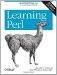# Section 4.6. Variable-Length Parameter Lists

### 4.6. Variable-Length Parameter Lists

In real-world Perl code, subroutines are often given parameter lists of arbitrary length. That's because of Perl's "no unnecessary limits" philosophy. Of course, this is unlike many traditional programming languages, which require every subroutine to be strictly typed to permit only a certain, predefined number of parameters of predefined types. It's nice that Perl is so flexible, but (as you saw with the &max routine earlier) that may cause problems when a subroutine is called with a different number of arguments than the author expected.

Of course, the subroutine can easily check that it has the right number of arguments by examining the @_ array. For example, we could have written &max to check its argument list like this:[*]

[*] As soon as you learn about warn in the next chapter, you'll see that you can use it to turn improper usage like this into a proper warning. Or perhaps you'll decide this case is severe enough to warrant using die, described in the same chapter.

`     sub max {       if (@_ != 2) {         print "WARNING! &max should get exactly two arguments!\n";       }       # continue as before...       .       .       .     }`

That if test uses the "name" of the array in a scalar context to find out the number of array elements, as you saw in Chapter 3.

But in real-world Perl programming, this sort of check is rarely used; it's better to make the subroutine adapt to the parameters.

#### 4.6.1. A Better &max Routine

So let's rewrite &max to allow for any number of arguments:

`     \$maximum = &max(3, 5, 10, 4, 6);     sub max {       my(\$max_so_far) = shift @_;  # the first one is the largest yet seen       foreach (@_) {               # look at the remaining arguments         if (\$_ > \$max_so_far) {    # could this one be bigger yet?           \$max_so_far = \$_;         }       }       \$max_so_far;     }`

This code uses what has often been called the high-water mark algorithm: after a flood, when the waters have surged and receded for the last time, the high-water mark shows where the highest water was seen. In this routine, \$max_so_far keeps track of our high-water mark, the largest number yet seen.

The first line sets \$max_so_far to 3 (the first parameter in the example code) by shifting that parameter from the parameter array, @_. So, @_ now holds (5, 10, 4, 6) since the 3 has been shifted off. The largest number yet seen is the only one yet seen: 3, the first parameter.

Now, the foreach loop will step through the remaining values in the parameter list from @_. The control variable of the loop is, by default, \$_. (But, remember, there's no automatic connection between @_ and \$_; it's a coincidence that they have similar names.) The first time through the loop, \$_ is 5. The if test sees that it is larger than \$max_so_far, so \$max_so_far is set to 5, which is the new high-water mark.

The next time through the loop, \$_ is 10. That's a new record high, so it's stored in \$max_so_far as well.

The next time, \$_ is 4. The if test fails since that's no larger than \$max_so_far, which is 10, so the body of the if is skipped.

The next time, \$_ is 6, and the body of the if is skipped again. And that was the last time through the loop, so the loop is done.

Now, \$max_so_far becomes the return value. It's the largest number we've seen, and we've seen them all, so it must be the largest from the list: 10.

#### 4.6.2. Empty Parameter Lists

That improved &max algorithm works fine now, even if there are more than two parameters. But what happens if there are none?

At first, it may seem too esoteric to worry about. After all, why would someone call &max without giving it any parameters? But maybe someone wrote a line like this one:

`     \$maximum = &max(@numbers);`

And the array @numbers might sometimes be an empty list; perhaps it was read in from a file that turned out to be empty. So you need to know this: What does &max do in that case?

The first line of the subroutine sets \$max_so_far by using shift on @_, the (now empty) parameter array. That's harmless; the array is left empty, and shift returns undef to \$max_so_far.

Now the foreach loop wants to iterate over @_, but since that's empty, the loop body is executed zero times.

In short order, Perl returns the value of \$max_so_farundefas the return value of the subroutine. In some sense, that's the right answer because there is no largest value in an empty list.

Of course, whoever is calling this subroutine should be aware that the return value may be undef, or they could ensure that the parameter list is never empty.Learning Perl, 5th Edition
ISBN: 0596520107
EAN: 2147483647
Year: 2003
Pages: 232

Similar book on Amazon

flylib.com © 2008-2017.
If you may any questions please contact us: flylib@qtcs.net